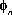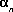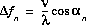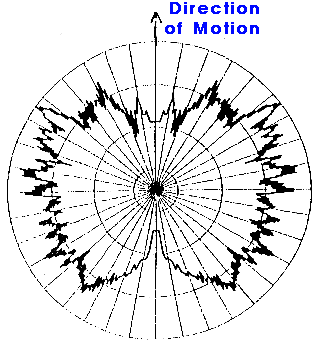### JPL's Wireless Communication Reference Website# Doppler spectrum

## Doppler shifts

Motion of an antenna produces Doppler shifts of incoming received waves. We consider a signal received over a multipath channel, with many incoming waves. Let the n-th reflected wave with amplitude cn and phasearrive from an anglerelative to the direction of the motion of the antenna.The Doppler shift of this wave is,

where v is the speed of the antenna.

The maximum Doppler shift occurs for a wave coming from the opposite direction as the direction the antenna is moving to. It has a frequency shift
 ``` v fm = -- fc c ```with fc is the carrier frequency and c the velocity of light.

Such motion of the antenna leads to (time varying) phase shifts of individual reflected waves. It is not so much this minor shift that bothers radio system designers, as a receiver oscillator can easily compensate for it. Rather, it is the fact that many waves arrive, all with different shifts. Thus, their relative phases change all the time, and so it affects the amplitude of the resulting composite signal. So the Doppler effects determine the rate at which the amplitude of the resulting composite signal changes.## Doppler Power Spectrum

The models behind Rayleigh or Rician fading assume that many waves arrive each with its own random angle of arrival (thus with its own Doppler shift), which is uniformly distributed within [0, 2p ], independently of other waves. This allows us to compute a probability density function of the frequency of incoming waves. Moreover, we can obtain the Doppler spectrum of the received signal.

Figure (on the right): Distribution of angle of arrival in a typical urban propagation situation. Measurement at 1800 MHz. Source: Research group of Prof. Paul Walter Baier, U. of Kaiserslautern, Germany.

This leads to the U-shaped power spectrum for isotropic scattering,

```                      1               1
S(f)  =  --------  ---------------------
4 p  fm                (f-fc)2
sqrt( 1 -   -------)
fm2
```
where we assumed a unity local mean power.Figure: Power density spectrum of a sine wave suffering from a Doppler spread.Figure: Measured Doppler spread at 1800 MHz. Doppler spread = 60.3 Hz Source: Research group of Prof. Paul Walter Baier, U. of Kaiserslautern, Germany. See also: full scatter plot.

If a sinusoidal signal is transmitted (represented by a spectral line in the frequency domain), after transmission over a fading channel, we will receive a power spectrum that is spread according to the above image. The frequency range where the power spectrum is nonzero defines the Doppler spread.

The Doppler spread is relevant, for instance to compute threshold crossing rates and average fade durations.

## How do systems handle Doppler Spreads?

 System Countermeasure Analog Doppler causes random FM modulation which may be audible. Carrier frequency is low enough to avoid problems GSM Channel bit rate well above Doppler spread TDMA during each bit / burst transmission the channel is fairly constant. Receiver training/updating during each transmission burst Feedback frequency correction DECT Intended for pedestrian use: Only small Doppler spreads are to be anticipated Bit rate is very large compared to Doppler spread. IS95 Cellular CDMA Downlink: Pilot signal for synchronization and channel estimation Uplink: Continuous tracking of each signal Wireless LAN's Mobility is slow, thus Doppler spread is only a few Hertz

## Effect of angle of arrival and excess delay

There are two different forms of multipath scattering, according to the excess time delay of the given channel tap:
1. Small Excess Time Delays
The channel tap may be modelled as the accumulation of multipath components received from scatters close to the mobile. This gives rise to the classical Doppler power spectrum of the received multi- path components.

2. Large Excess Time Delays
The classical Doppler model does not provide a satisfactory geometric model for this type of scattering. Instead, multipath energy is more likely to have a narrow Doppler spread, having arisen from reflec- tions off isolated obstacles such as buildings or hills. The instantaneous variation of signal power in space for a channel depends on the angles of arrival of the multipath components.